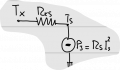# Temperature and Voltage of an NTC sensor

#### kalemaxon89

Joined Oct 12, 2022
158
I have an object 'x' with its own temperature Tx and a NTC sensor 's' with:
- its own temperature Tx [°C]
- polarised with a current Is [A]
- infinite thermal capacity Cs (consider the object as a generator of V and not influenced by the self heating Pj)
- a self heating Pj
Between the sensor and the object there is a thermal resistance Rxs [°C/W]In the thermal model, temperature is the analogue of electric voltage, heat flow rate is the analogue of current, etc. .. and in this example you have to solve (if I am not mistaken) a circuit with V, I and R ... nothing more.

Without discussing and listing what needs to be found etc., I am interested in this particular:
on the notes taken in class, the temperature difference deltaT between Ts and Tx calculated as follows: Rxs*Rs*I^2 .. why?
Should it be simply Rxs*Is? :/

Last edited:

#### WBahn

Joined Mar 31, 2012
28,469
I have an object 'x' with its own temperature Tx and a NTC sensor 's' with:
- its own temperature Tx
-
polarised with a current Is
-
infinite thermal capacity Cs (consider the object as a generator of V and not influenced by the self heating Pj)
- a self heating Pj
Between the sensor and the object there is a thermal resistance Rxs.

View attachment 290618

In the thermal model, temperature is the analogue of electric voltage, heat flow rate is the analogue of current, etc. .. and in this example you have to solve (if I am not mistaken) a circuit with V, I and R ... nothing more.

Without discussing and listing what needs to be found etc., I am interested in this particular:
on the notes taken in class, the temperature difference deltaT between Ts and Tx calculated as follows: Rxs*Rs*I^2 .. why?
Should it be simply Rxs*Is? :/
What are the units on thermal resistance?

Will multiplying a thermal resistance by a current yield a temperature difference?

#### kalemaxon89

Joined Oct 12, 2022
158
What are the units on thermal resistance?

Will multiplying a thermal resistance by a current yield a temperature difference?
Just updated the post with units of measurement.
Anyway, resistance Rxs [°C/W]

#### WBahn

Joined Mar 31, 2012
28,469
Just updated the post with units of measurement.
Anyway, resistance Rxs [°C/W]
And what do you get if you multiply this by a current, like you are suggesting?

What type of quantity do you need to multiply Rxs by in order to be left with units of temperature?